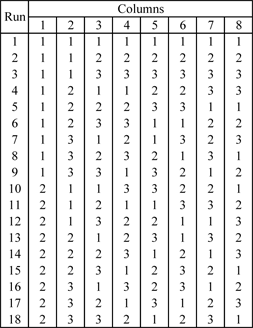# Taguchi Orthogonal Arrays

 Appendix D Taguchi Orthogonal Arrays

## Two Level Designs

L4 (2^3)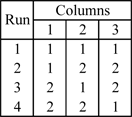L8 (2^7)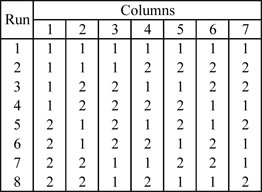L12 (2^11)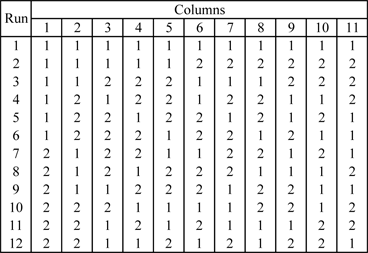L16 (2^15)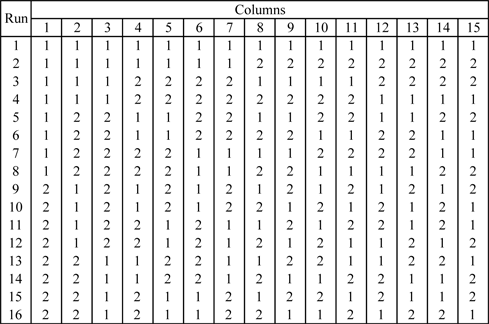## Three Level Designs

L9 (3^4)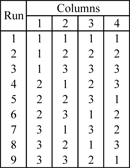L27 (3^13)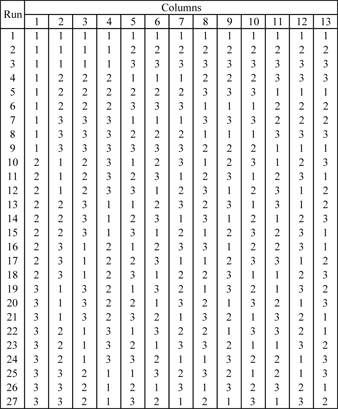## Mixed Level Designs

L8 (2^4 4^1)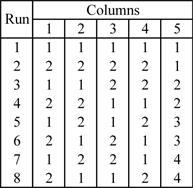L16 (2^12 4^1)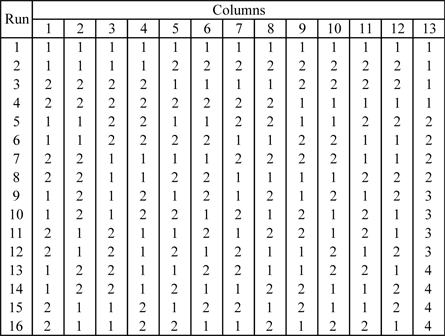L16 (2^9 4^2)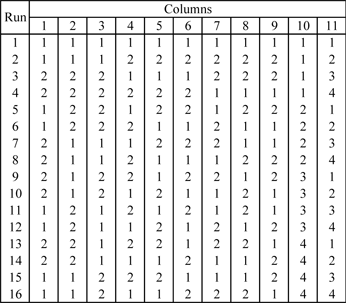L16 (2^6 4^3)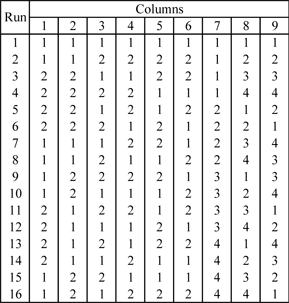L16 (2^3 4^4)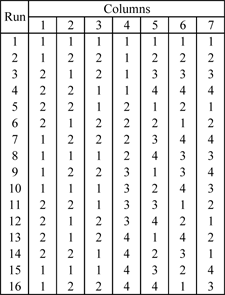L18 (2^1 3^7)